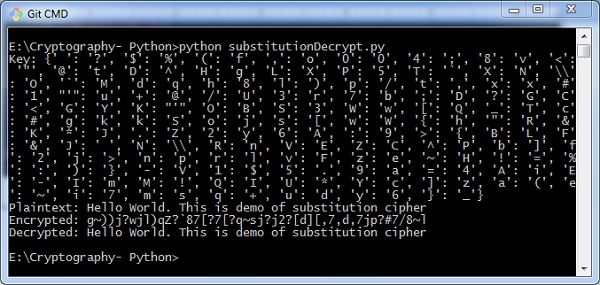# Decryption of Simple Substitution Cipher

In this chapter, you can learn about simple implementation of substitution cipher which displays the encrypted and decrypted message as per the logic used in simple substitution cipher technique. This can be considered as an alternative approach of coding.

## Code

You can use the following code to perform decryption using simple substitution cipher −

```import random
chars = 'ABCDEFGHIJKLMNOPQRSTUVWXYZ' + \
'abcdefghijklmnopqrstuvwxyz' + \
'0123456789' + \
':.;,?!@#\$%&()+=-*/_<> []{}`~^"\'\\'

def generate_key():
"""Generate an key for our cipher"""
shuffled = sorted(chars, key=lambda k: random.random())
return dict(zip(chars, shuffled))

def encrypt(key, plaintext):
"""Encrypt the string and return the ciphertext"""
return ''.join(key[l] for l in plaintext)

def decrypt(key, ciphertext):
"""Decrypt the string and return the plaintext"""
flipped = {v: k for k, v in key.items()}
return ''.join(flipped[l] for l in ciphertext)

def show_result(plaintext):
"""Generate a resulting cipher with elements shown"""
key = generate_key()
encrypted = encrypt(key, plaintext)
decrypted = decrypt(key, encrypted)

print 'Key: %s' % key
print 'Plaintext: %s' % plaintext
print 'Encrypted: %s' % encrypted
print 'Decrypted: %s' % decrypted
show_result('Hello World. This is demo of substitution cipher')```

## Output

The above code gives you the output as shown here −